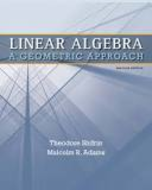### Solution Found!# Find the general solution of each of the following equations (presented, as in the text

Chapter 1, Problem 3

(choose chapter or problem)

Get Unlimited Answers! Check out our subscriptions
QUESTION:

Find the general solution of each of the following equations (presented, as in the text, as a combination of an appropriate number of vectors)

a. $$x_{1}-2 x_{2}+3 x_{3}=4\left(\text { in } \mathbb{R}^{3}\right)$$

b. $$x_{1}+x_{2}-x_{3}+2 x_{4}=0\left(\text { in } \mathbb{R}^{4}\right)$$

c. $$x_{1}+x_{2}-x_{3}+2 x_{4}=5\left(\text { in } \mathbb{R}^{4}\right)$$

d. $$x_{1}-2 x_{2}+3 x_{3}=4\left(\text { in } \mathbb{R}^{4}\right)$$

e. $$x_{2}+x_{3}-3 x_{4}=2\left(\text { in } \mathbb{R}^{4}\right)$$

### Questions & Answers (3 Reviews)

QUESTION:

Find the general solution of each of the following equations (presented, as in the text, as a combination of an appropriate number of vectors)

a. $$x_{1}-2 x_{2}+3 x_{3}=4\left(\text { in } \mathbb{R}^{3}\right)$$

b. $$x_{1}+x_{2}-x_{3}+2 x_{4}=0\left(\text { in } \mathbb{R}^{4}\right)$$

c. $$x_{1}+x_{2}-x_{3}+2 x_{4}=5\left(\text { in } \mathbb{R}^{4}\right)$$

d. $$x_{1}-2 x_{2}+3 x_{3}=4\left(\text { in } \mathbb{R}^{4}\right)$$

e. $$x_{2}+x_{3}-3 x_{4}=2\left(\text { in } \mathbb{R}^{4}\right)$$

Step 1 of 3

a)Because there are variables in this one equation,specifying two determines the third.So let’s set two of them equal to arbitrary variable names.

Let $$x_{2}=s$$

and $$x_{3}=t$$

Then we see that $$x_{1}-x_{2}+3 x_{3}=4=x_{1}=4+2 s-3 t$$.

Thus the solution set is all vectors of the form

$$X=\left(x_{1}, x_{2}, X-3\right)=(4+2 s-3 t, s, t)=(4,0,0)+s(2,1,0)+t(-3,0,1)$$

b)

In the rest of these we proceed exactly in (a).

So Let $$x_{2}=r$$

$$x_{3}=s$$

and $$x_{4}=t$$.

Then

$$x=(-r+s-2 t, r, s, t)=r(-1,1,0,0)+s(1,0,1,0)+t(-2,0,0,1)$$

### Review this written solution for 964024) viewed: 285 isbn: 9781429215213 | Linear Algebra: A Geometric Approach - 2 Edition - Chapter 1.3 - Problem 3

Thank you for your recent purchase on StudySoup. We invite you to provide a review below, and help us create a better product.

Textbook: Linear Algebra: A Geometric Approach

Click to rate

Write a review below (optional):

### Review this written solution for 964024) viewed: 285 isbn: 9781429215213 | Linear Algebra: A Geometric Approach - 2 Edition - Chapter 1.3 - Problem 3

Thank you for your recent purchase on StudySoup. We invite you to provide a review below, and help us create a better product.

Textbook: Linear Algebra: A Geometric Approach

Click to rate

Write a review below (optional):

### Review this written solution for 964024) viewed: 285 isbn: 9781429215213 | Linear Algebra: A Geometric Approach - 2 Edition - Chapter 1.3 - Problem 3

Thank you for your recent purchase on StudySoup. We invite you to provide a review below, and help us create a better product.

Textbook: Linear Algebra: A Geometric Approach

Click to rate

Write a review below (optional):